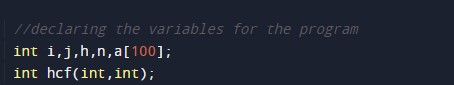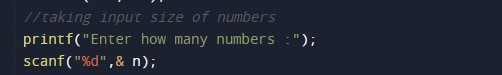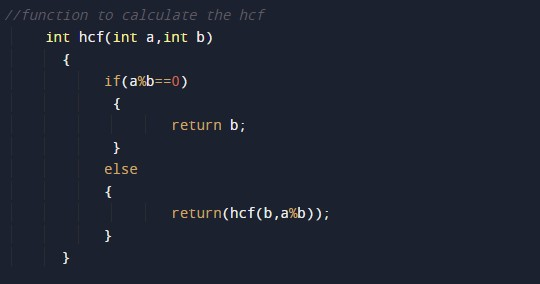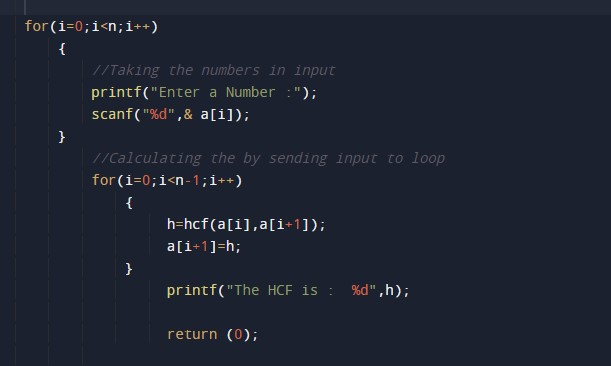C Program to Find HCF of n Numbers

In this tutorial you will learn about the C Program to Find HCF of n Numbers and its application with practical example.

C Program to Find HCF of n Numbers

In this tutorial, we will learn to create a C program that will Find HCF of n numbers using C programming.

Prerequisites

Before starting with this tutorial we assume that you are best aware of the following C programming topics:

• Operators in C Programming.
• Basic Input and Output function in C Programming.
• Basic C programming.
• For loop in C programming.
• Arithmetic operations in C Programming.

Program to Find HCFof n numbers

In c programming, it is possible to take numerical input from the user and find HCF of n numbers with the help of a very small amount of code. The C language has many types of header libraries which has supported function in them with the help of these files the programming is easy.

With the help of this program, we can Find HCF of n numbers

Here,

HCF:- Highest Common Factor.

Output:-In the above program, we have first initialized the required variable.• i = it will hold the integer value.
• j = it will hold the integer value.
• h = it will hold the integer value.
• n = it will hold the integer value.
• a = it will hold the integer value.

Input number from the user.User-defined function HCF.Input-output code in the main method body.In this tutorial we have learn about the C Program to Find HCF of n Numbers and its application with practical example. I hope you will like this tutorial.